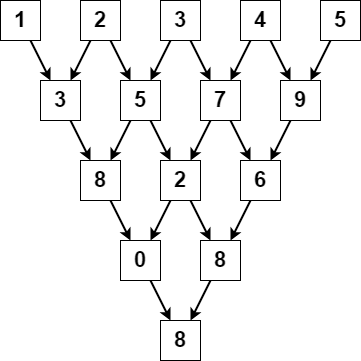##### Welcome to Subscribe On Youtube

Formatted question description: https://leetcode.ca/all/2221.html

# 2221. Find Triangular Sum of an Array (Medium)

You are given a 0-indexed integer array nums, where nums[i] is a digit between 0 and 9 (inclusive).

The triangular sum of nums is the value of the only element present in nums after the following process terminates:

1. Let nums comprise of n elements. If n == 1, end the process. Otherwise, create a new 0-indexed integer array newNums of length n - 1.
2. For each index i, where 0 <= i < n - 1, assign the value of newNums[i] as (nums[i] + nums[i+1]) % 10, where % denotes modulo operator.
3. Replace the array nums with newNums.
4. Repeat the entire process starting from step 1.

Return the triangular sum of nums.

Example 1:Input: nums = [1,2,3,4,5]
Output: 8
Explanation:
The above diagram depicts the process from which we obtain the triangular sum of the array.

Example 2:

Input: nums = 
Output: 5
Explanation:
Since there is only one element in nums, the triangular sum is the value of that element itself.

Constraints:

• 1 <= nums.length <= 1000
• 0 <= nums[i] <= 9

Similar Questions:

## Solution 1. Pascal’s Triangle

• // OJ: https://leetcode.com/problems/find-triangular-sum-of-an-array/
// Time: O(N^2)
// Space: O(1) extra space
class Solution {
public:
int triangularSum(vector<int>& A) {
for (int i = A.size(); i >= 1; --i) {
for (int j = 0; j < i - 1; ++j) {
A[j] = (A[j] + A[j + 1]) % 10;
}
}
return A;
}
};

• class Solution:
def triangularSum(self, nums: List[int]) -> int:
n = len(nums)
for i in range(n, 0, -1):
for j in range(i - 1):
nums[j] = (nums[j] + nums[j + 1]) % 10
return nums

############

# 2221. Find Triangular Sum of an Array
# https://leetcode.com/problems/find-triangular-sum-of-an-array/

class Solution:
def triangularSum(self, nums: List[int]) -> int:
n = len(nums)

for _ in range(n - 1):
curr = []

for i in range(1, len(nums)):
curr.append((nums[i - 1] + nums[i]) % 10)

nums = curr

return nums


• class Solution {
public int triangularSum(int[] nums) {
int n = nums.length;
for (int i = n; i >= 0; --i) {
for (int j = 0; j < i - 1; ++j) {
nums[j] = (nums[j] + nums[j + 1]) % 10;
}
}
return nums;
}
}

• func triangularSum(nums []int) int {
n := len(nums)
for i := n; i >= 0; i-- {
for j := 0; j < i-1; j++ {
nums[j] = (nums[j] + nums[j+1]) % 10
}
}
return nums
}


## Discuss

https://leetcode.com/problems/find-triangular-sum-of-an-array/discuss/1907038/